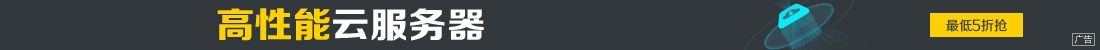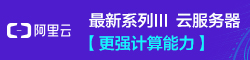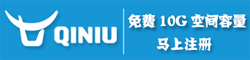# php依赖注入知识点详解

••php中传递依赖关系的三种方案

```<?php

class A

{

public function __construct()

{

\$b = new B();

}

}```

```<?php

class A

{

public function __construct(B \$b)

{

}

}```

```<?php

class A

{

public function setB(B \$b)

{

\$this->b = \$b;

}

}```

（或者说一个容器）可以帮我们管理这些依赖关系就好了。

```<?php

class Container {

private \$s=array();

function __set(\$k, \$c) { \$this->s[\$k]=\$c; }

function __get(\$k) { return \$this->s[\$k](\$this); }

}```

```<?php

class A

{

private \$container;

public function __construct(Container \$container)

{

\$this->container = \$container;

}

public function doSomeThing()

{

//do something which needs class B

\$b = \$this->container->getB();

//to do

}

}```

```\$c = new Container();

\$c->setB(new B());```

```\$c = new Container();

\$c->setB(function (){

return new B();

});```